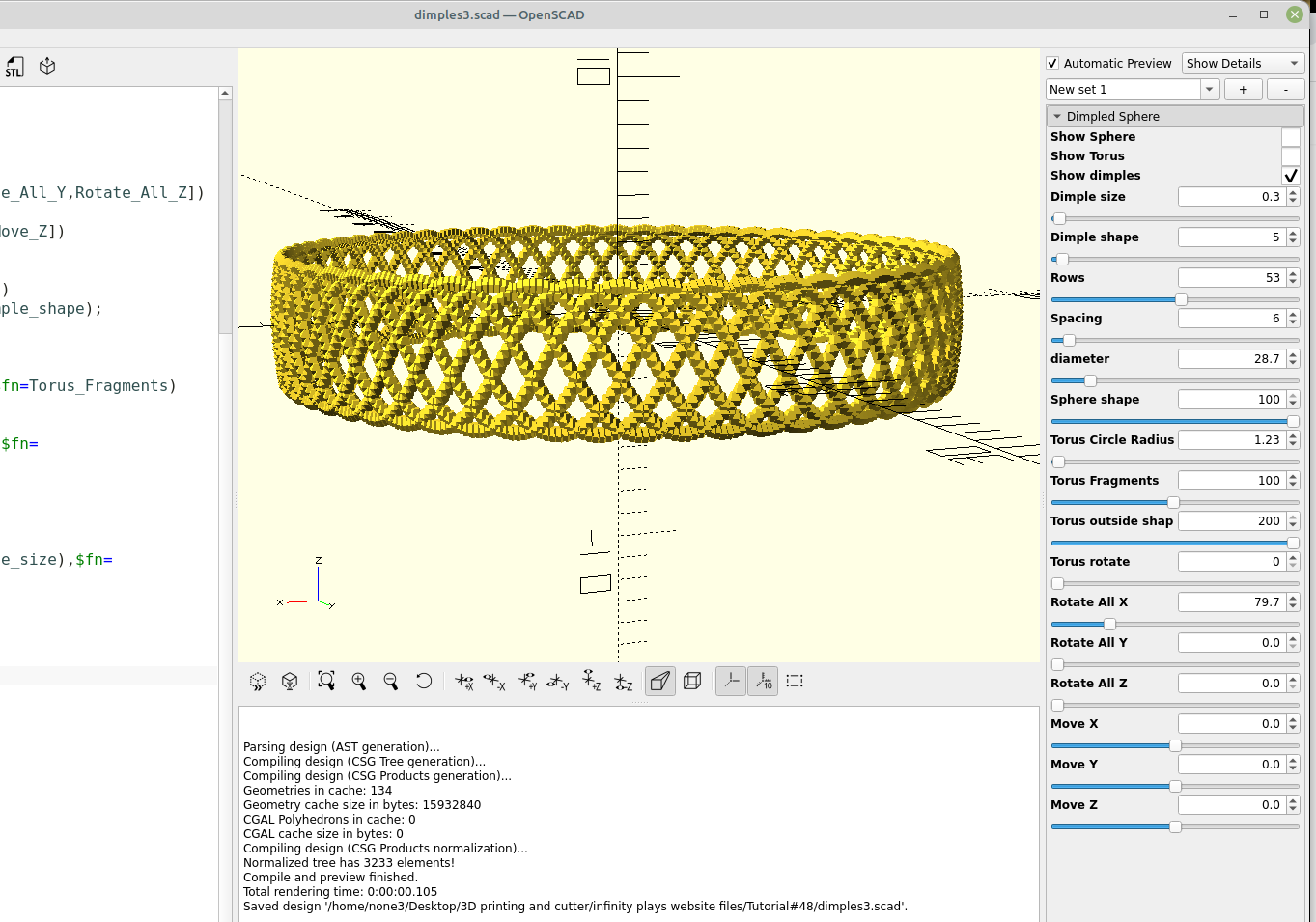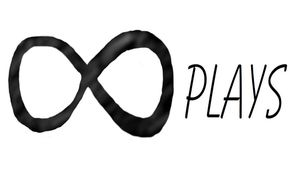By Bob

Just for fun I wanted to make a module that could put dimples or bumps on different shaped surfaces, I was able to recycle the code from other modules and come up with a few ideas. I will definitely be making improvements and adding features to this.

Here is the code:

``````/* [Dimpled Sphere] */
Show_Sphere = true;
Show_Torus = false;
Show_dimples = false;
Dimple_size = .5; //[.1:.1:20]
Dimple_shape = 30;//[3:1:100]
Rows = 20; //[1:1:100]
Spacing = 15;//[1:1:100]
diameter = 30; //[1:.1:200]
Sphere_shape = 30;//[3:1:100]
Torus_Fragments = 100; //[3:1:200]
Torus_outside_shape =100; //[3:1:200]
Torus_rotate =45; //[0:1:360]
Rotate_All_X = 0; //[0:.1:360]
Rotate_All_Y = 0; //[0:.1:360]
Rotate_All_Z = 0; //[0:.1:360]
Move_X = 0; //[-200:.1:200]
Move_Y = 0; //[-200:.1:200]
Move_Z = 0; //[-200:.1:200]

module Dimples(){
rotate([Rotate_All_X,Rotate_All_Y,Rotate_All_Z])
rotate([90,0,0])
translate([Move_X,Move_Y,Move_Z])
for(h=[1:Rows])
rotate([0,0,h*360/Rows])
translate([0,diameter/2,0])
sphere(Dimple_size,\$fn=Dimple_shape);
}

module Torus(){
rotate_extrude(convexity=10,\$fn=Torus_Fragments)
translate([diameter/2, 0, 0])
rotate([0,0,Torus_rotate])
}

if(Show_Sphere)
difference(){
sphere(diameter/2-(.5*Dimple_size),\$fn=Sphere_shape);
for (R=[0:Spacing:360])
rotate([0,0,R])
Dimples();
}

if(Show_Torus)
difference(){
Torus();
for (R=[0:Spacing:360])
rotate([0,0,R])
Dimples();
}

if (Show_dimples){
for (R=[0:Spacing:360])
rotate([0,0,R])
Dimples();
}``````

Here are some dimples on a sphere. I wasn't trying to make a golf ball or anything in particular, I just wanted to see what it would look like.

I added a "show dimples" checkbox so you can see the dimples or make a design out of them to print:right click and open in new tab for a larger version

``````/* [Dimpled STL] */
Path_to_STL_File ="/home/none3/Desktop/YourFileName.stl";
Show_Difference = false;
Show_STL = true;
Dimple_size = .5; //[.1:.1:20]
Dimple_shape = 30;//[3:1:100]
Rows = 20; //[1:1:100]
Spacing = 15;//[1:1:100]
diameter = 30; //[1:.1:200]
Rotate_All_X = 0; //[0:.1:360]
Rotate_All_Y = 0; //[0:.1:360]
Rotate_All_Z = 0; //[0:.1:360]
Move_X = 0; //[-200:.1:200]
Move_Y = 0; //[-200:.1:200]
Move_Z = 0; //[-200:.1:200]

module Dimples(){
rotate([Rotate_All_X,Rotate_All_Y,Rotate_All_Z])
rotate([90,0,0])
translate([Move_X,Move_Y,Move_Z])
for(h=[1:Rows])
rotate([0,0,h*360/Rows])
translate([0,diameter/2,0])
sphere(Dimple_size,\$fn=Dimple_shape);
}

module DimpleStl(){
if(Show_Difference)
difference(){
import(Path_to_STL_File,convexity=11);
for (R=[0:Spacing:360])
rotate([0,0,R])
Dimples();
}
else{
if(Show_STL)
import(Path_to_STL_File,convexity=11);
for (R=[0:Spacing:360])
rotate([0,0,R])
Dimples();
}}

DimpleStl();``````

I highly recommend trying out the newest version of OpenScad,  I have been using the appimage on Linux and have had zero crashes and rendering times are impressive.

If you are interested in making a golf ball or Christmas ornaments here's some code to spiralize the dimples to space them more evenly over the surface of the sphere:

``````Show_bumps = false;
Show_dimples = true;
Spiralize = .129; //[.01:.001:.190]
Randomize = .738; //[.01:.001:1]
Dispersion = 2; //[.01:.01:2]
Bump_size = .50; // [.01:.01:3]
Bump_shape = 50; //[3:1:100]
Bump_number=307; //[1:.01:1000]
Sphere_size = 10; //[1:.01:100]
Shell_thickness = .90; //[.90:.01:1]

module Dimples(){
for(i=[0:Bump_number]) {
theta = 360*i/(Spiralize/Randomize);
phi = acos(1-Dispersion*i/Bump_number);
translate([x,y,z])
sphere(Bump_size,\$fn=Bump_shape);
}}

if(Show_dimples)
difference() {
sphere(Sphere_size,\$fn=160);
sphere(Sphere_size*Shell_thickness);
Dimples();
}

if (Show_bumps)
Dimples();``````

## Related ArticlesFollow